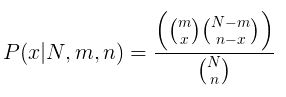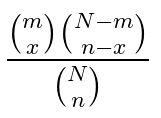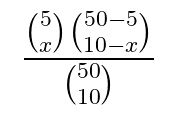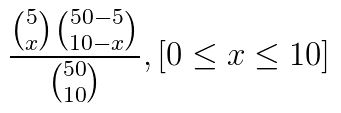# Hypergeometric Distribution Formula

Hypergeometric distribution is a random variable of a hypergeometric probability distribution. Using the formula of you can find out almost all statistical measures such as mean, standard deviation, variance etc.Where,

N: The number of items in the population.
n: The number of items in the sample.
x: The number of items in the sample that are classified as successes.
P(x| N, n, k): hypergeometric probability – the probability that an n-trial hypergeometric experiment results in exactly x successes, when the population consists of

$$\begin{array}{l}N\end{array}$$
items,
$$\begin{array}{l}k\end{array}$$
of which are classified as successes.

### Solved Examples

Question 1: Calculate the probability density function of the hypergeometric function if N, n and m are 50, 10 and 5 respectively ?

Solution:
Given parameters are,
N = 50
n = 10
m = 5

Formula for hypergeometric distribution is,

P(x|N,m,n) =P(x|N,m,n) =So, the probability distribution function is,

P(x|50, 5, 10) =